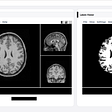# Understanding the Frenet-Serret frame

• Sensor reading in the inertial(or sensor) frame
• The path the vehicle needs to follow. This path is nothing but an array of equidistant points( x and y coordinates) in the vehicle frame.
1. Get the sensor reading and the path points in the same frame. Here, we will bring them in the vehicle frame, since the transformation between vehicle and sensor frame is known and will remain constant.
2. Find a point on the path, such that if you join the obstacle center with that point, the line will be perpendicular to the tangent. Or in other words, find the point on the path nearest to the obstacle center. (If you are confused at this point, carefully look the figure again). Let’s call this as point C.
3. Find a point immediately next (point N) and immediately prior (point P) to the point from step (1). If we know the index of point C, then point N will be index + 1 and point P will be index -1.
4. Here we will be approximating some quantities. Since the points are equidistant, (N-P) will result in a vector that is almost tangent to point C. Let’s call this as vector T.
5. Next, we will subtract point P from the obstacle center to obtain vector V1. (refer figure if you are lost).
6. Now comes the vector algebra. Somehow, we need to find the value of d. To do so, we will perform a cross-product operation on vector V1 and vector T. The magnitude of the resultant will be |V1||T|sinΘ. By dividing the term by |T|, I will get |V1|sinΘ, which is nothing but the projection of vector V1 on vector T. I am calling this quantity as an approximated value of d. If you disagree with me, refer to the below image.

--

--

--

## More from Sakshi Kakde

Aspiring Roboticist. ❤️ to explore

Love podcasts or audiobooks? Learn on the go with our new app.

## How To Create Your Own Writing Assistant App Using Python## Statistical Learning (V): Unsupervised Learning## Building a Covid19 mask detector with OpenCV, Keras and Tensorflow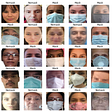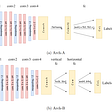## Fashion-MNIST using Machine Learning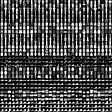## My Introduction to Transfer Learning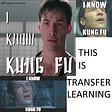## Affine Transformation- Image Processing In TensorFlow- Part 1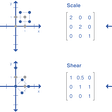## Naive Bayes & its Mathematical Implementation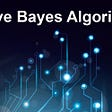## Sakshi Kakde

Aspiring Roboticist. ❤️ to explore

## Slime Robot! Why isn’t anyone talking about it?## The change in jobs because of robots## CoreDump: PCoIP Zero Client based robotics.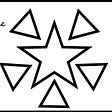## Brainchop: In-browser 3D MRI Segmentation